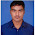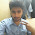VHDL coding tips and tricks: 4 bit comparator with testbench

## Sunday, March 28, 2010

### 4 bit comparator with testbench

Here is the code for 4 bit comparator using if .. elsif ... else statements.The module has two 4-bit inputs which has to be compared, and three 1-bit output lines.One of these output lines goes high depending upon whether the first number is equal to,less or greater than the second number.

--libraries to be used are specified here
library IEEE;
use IEEE.STD_LOGIC_1164.ALL;

--entity declaration with port definitions
entity compare is
port(    num1 : in std_logic_vector(3 downto 0);  --input 1
num2 :      in std_logic_vector(3 downto 0);  --input 2
less :              out std_logic;   -- indicates first number is small
equal :          out std_logic;   -- both are equal
greater :   out std_logic    -- indicates first number is bigger
);
end compare;

--architecture of entity
architecture Behavioral of compare is

begin
process(num1,num2)
begin    -- process starts with a 'begin' statement
if (num1 > num2 ) then  --checking whether num1 is greater than num2
less <= '0';
equal <= '0';
greater <= '1';
elsif (num1 < num2) then    --checking whether num1 is less than num2
less <= '1';
equal <= '0';
greater <= '0';
else     --checking whether num1 is equal to num2
less <= '0';
equal <= '1';
greater <= '0';
end if;
end process;   -- process ends with a 'end process' statement

end Behavioral;

The test bench program used for testing the design is given below:

library IEEE;
use IEEE.STD_LOGIC_1164.ALL;

--this is how entity for your test bench code has to be declared.
entity testbench is
end testbench;

architecture behavior of testbench is
--signal declarations.
signal num1,num2 : std_logic_vector(3 downto 0) :=(others => '0');
signal less,equal,greater :  std_logic:='0';

begin
--entity instantiation
UUT : entity work.compare port map(num1,num2,less,equal,greater);

--definition of simulation process
tb : process
begin
num1<="0010";  --num1 =2
num2<="1001"; --num2 =9
wait for 2 ns;

num1<="1001";  --num1 =9
num2<="0010";   --num2 =2
wait for 2 ns;

num1<="1010";  --num1 =10
num2<="1010";  --num2 =10
--more input combinations can be given here.
wait;
end process tb;

end;

The simulated waveform is shown below:

The code was synthesized using XILINX ISE XST . The RTL schematic of the design is shown below:

Note :- Use RTL Viewer to get a closer look on how your design is implemented in hardware.

#### 6 comments:

1.Hi i need same program for one 24 bit to be compared with hex value FAF320

2.very clear...i liked it...:)

3.plz compare sine & triangular like this!

4.compare sine & triangular!

5.can any 1 please help me out.is there any code for scalable digital cmos comparator using a parallel prefix tree.xilinx
please mail me mithra4444@gmail.com

6.please help me with the code for steganography of lsb algorithm